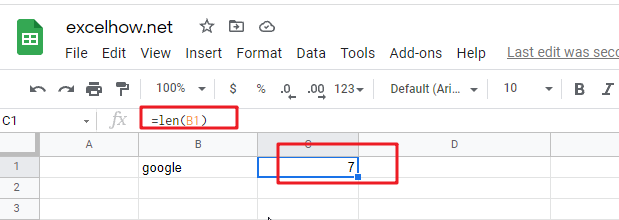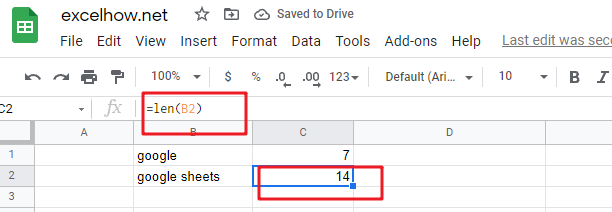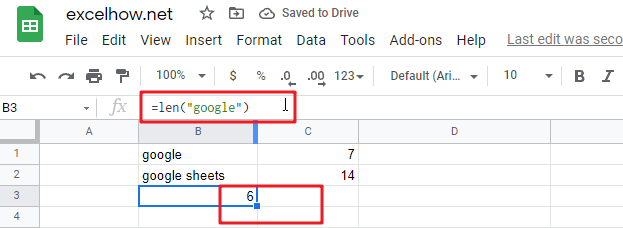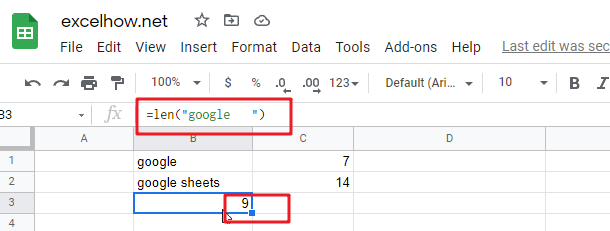# ExcelHow

This post will guide you how to use Google Sheets LEN function with syntax and examples.

## Description

The Google Sheets LEN function returns the length of a text string (the number of characters in a text string).

The LEN function can be used to count the number of characters for a given text string in google sheets. It also can count characters in numbers, but it will not count the number formatting.

The LEN function is a build-in function in Google Sheets and it is categorized as a Text Function.

## Syntax

The syntax of the LEN function is as below:

= LEN(text)

Where the LEN function arguments are:

• Text -This is a required argument. The text string that you want to get the length of.

Note:

• The LEN function only takes one argument. It counts the number of characters in text string, including space or non-printing characters. If the LEN function returns unexpected values, and you need to make sure that there are no such characters in given text string.
• If text string is an empty string or cell reference to an empty cell, the LEN function returns
• Len function will also count the characters in numeric value

## Google Sheets LEN Function Examples

The below examples will show you how to use google sheets LEN Text function to get the length of a text string.

#1 To get the number of characters in the B1 cell, just using formula:

`=LEN(B1)`#2 To get the Length of the text string in B2 Cell, just using formula:

`=LEN(B2)`#3 Using LEN function to count the number of characters in a text string:

`=LEN(“google”)   //it returns 6`#4 count the number of characters in a text string in which contain a space character:

`=LEN(“google      “)  //it returns 9`The LEN function can be used with the LEFT function and FIND function to extract a substring text to the left of a given text string .